#### definition

Quadrilaterals: A four-sided polygon is a quadrilateral. Any four points in a plane, out of which three are non-collinear are joined in order to formed a four-sided closed figure called ‘quadrilateral’.

#### notes

• Any four points in a plane, out of which three are non-collinear are joined in order to formed a four-sided closed figure called ‘quadrilateral’.
• A quadrilateral has four sides, four angles and four vertices. Quadrilateral could be regular or irregular.
• A four-sided polygon is a quadrilateral.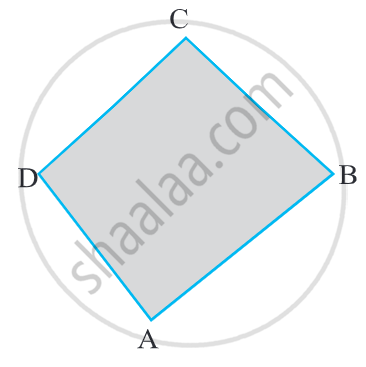• Quadrilateral ABCD has four sides bar"AB", bar"BC", bar"CD", and bar"DA".
• It has four angles ∠A, ∠B, ∠C, and ∠D.
• A, B, C and D are the four vertices and
• BD and AC are the two diagonals of the quadrilateral ABCD.

• A quadrilateral can be named by starting at any vertex and going serially either clockwise or anti-clockwise around the figure.

• When writing the name of a quadrilateral a sign like this ‘□’ is put in place of the word ‘quadrilateral’.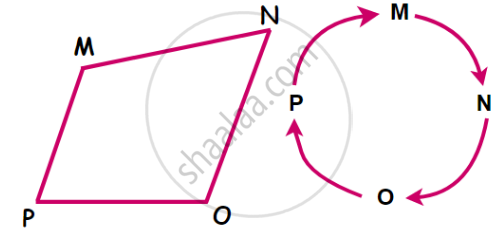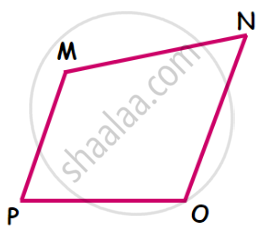• The sides MN and MP of □ MNOP have a common vertex M. Sides MN and MP are adjacent sides.

1. Side MN and Side MP

2. Side MN and Side NO

3. Side NO and Side OP

4. Side OP and Side MP.

## 2. Opposite Sides of a Quadrilateral: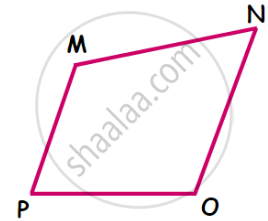• Opposite sides of the quadrilateral do not have a common vertex.
• In □  MNOP the sides MP and NO have no common vertex.
• Side MP and side NO are opposite sides of the quadrilateral.

### Pairs of opposite sides:

1. sides MP and NO

2. sides MN and PO• The angles of a quadrilateral which have one common arm are called adjacent angles of the quadrilateral.
• These angles are neighbouring or adjacent angles.

1. ∠MNO and ∠PMN

2. ∠MPO and ∠NOP

3. ∠PON and ∠MNO

4. ∠ONM and ∠PMN

## 4. Opposite Angles of a Quadrilateral:• The angles of a quadrilateral which do not have a common arm are called opposite angles of a quadrilateral.
• They lie opposite to each other.

### Pair of Opposite angle:

1. Angle opposite to ∠PMN is ∠NOP.
2. Angle opposite to ∠MNO is ∠OPM.

## 5. Diagonals of a Quadrilateral: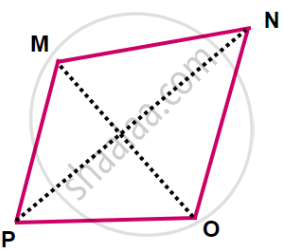• The line segments which join the vertices of the opposite angles of a quadrilateral are the diagonals of the quadrilateral.
• The segments MO and NP are the diagonals of the quadrilateral ABCD.

## 6. Interior & Exterior of A Quadrilateral: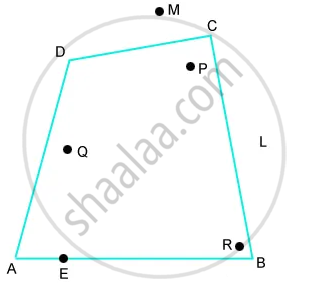• Being a polygon, a quadrilateral has an exterior and an interior.
• P, Q, and R are in the interior of the quadrilateral, M and L are in the exterior, and A, B, C, D, and E on the quadrilateral.
If you would like to contribute notes or other learning material, please submit them using the button below.

S
0%